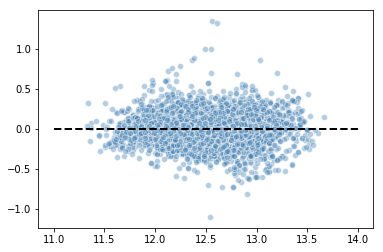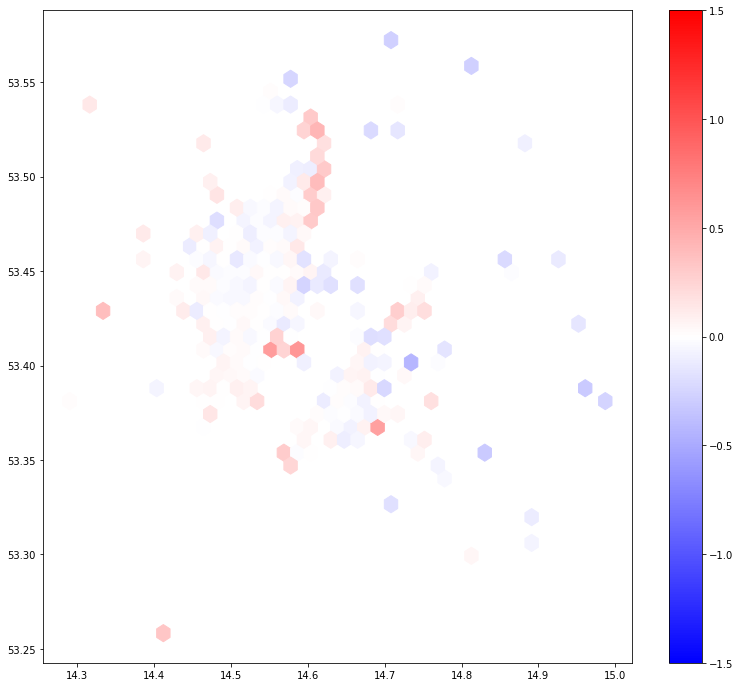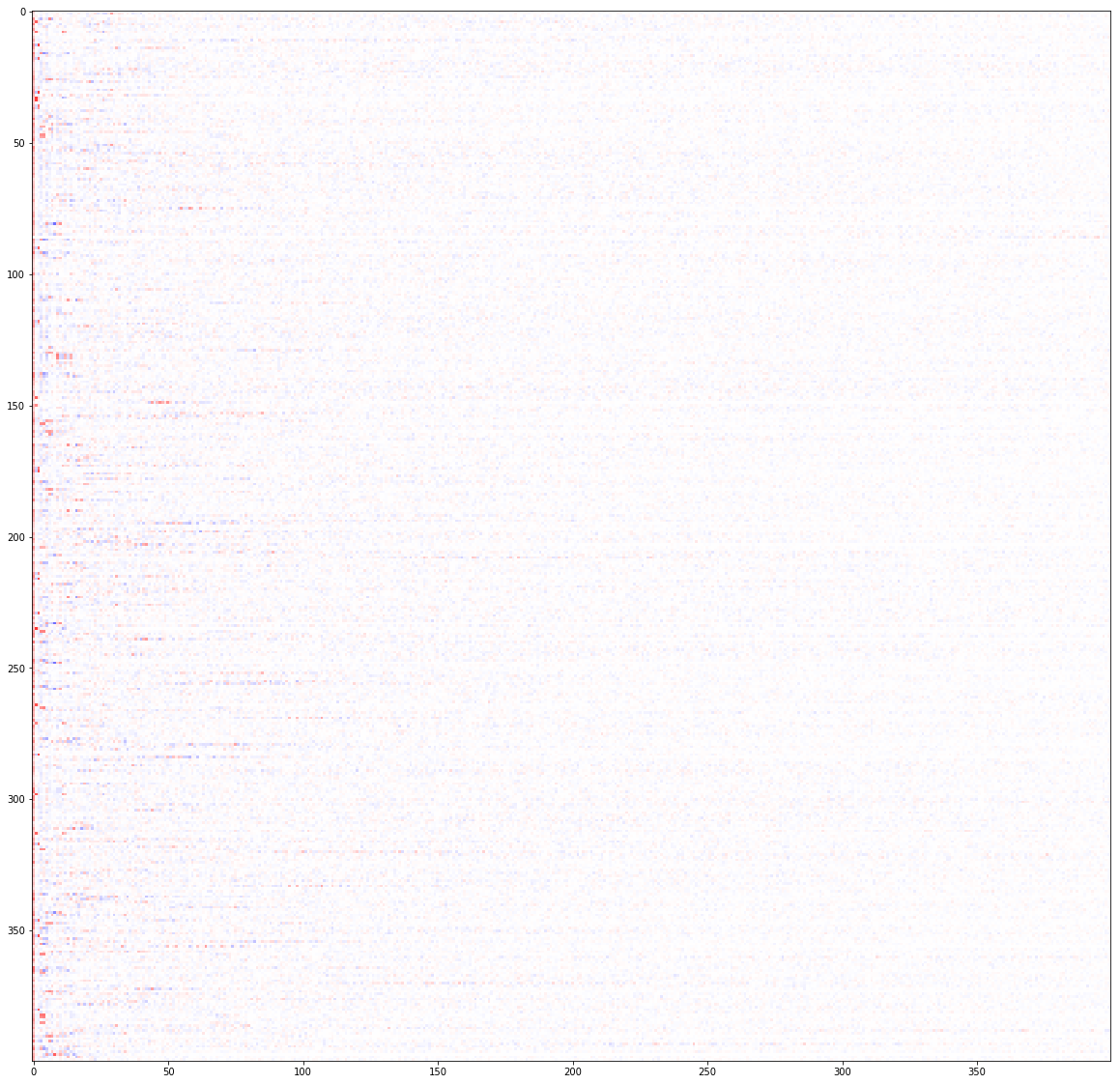# Housing data analysis using Machine Learning

In this entry I want to further expand my understanding of the Szczecin housing data which I analyzed preliminary in Szczecin housing market data introduction. It is exciting to see what you can do nowadays with data exploration and analysis. Since long I was thinking about expanding the simple OLS analysis of house prices in Szczecin by information available in the details and text descriptions of each offer.

At some point I got interested in machine learning. Somehow I find it more intuitive and results oriented than classical econometric methods. When I compare the two I see that econometric methods put more emphasis on understanding relationships between the data, while machine learning focuses on prediction efficiency. I like to view econometrics as knowledge generating process and machine learning as more fit for accurate out of sample predictions. Nevertheless knowledge gained while studying econometrics and applying econometric models helped me a lot to understand machine learning, because many of those methods overlap.

## ML books¶

I started my immersion with ML first by studying in depth Pandas library in Python. I used Python for Data Analysis: Data Wrangling with Pandas, NumPy, and IPython as my study material. I already had some understanding of NumPy from writing my master's thesis on protein unfolding couple of years ago. I must say that the material there is extremely comprehensive. I often return to this book whenever I need to understand better how a Pandas function works in greater detail. I also enjoyed the MatplotLib chapters. I have found them useful.

Next I turned to Hands-On Machine Learning with Scikit-Learn and TensorFlow: Concepts, Tools, and Techniques to Build Intelligent Systems. I find this book illuminating in terms of ML workflow and vocabulary. It presents a wide array of methods. It's a great primer into structuring the whole data analysis process. I greatly enjoyed these parts. What I didn't like about this book was that it was too fast. There were many topics covered and I felt that they were presented too quickly and without enough details to understand. Many articles on ML methods point out that it is part science, part art. I felt that this book laked the art part. I didn't enjoy the TensorFlow part at all. I felt rushed through it.

The most recent book that I went through was Python Machine Learning: Machine Learning and Deep Learning with Python, scikit-learn, and TensorFlow. I won't overstate that this book was a lifesaver for me! It's well paced and it has the perfect balance between breadth and depth. It's a typical vademecum where the author is like a passionate educator guiding the reader by hand through every aspect of the world of ML. The code examples are brilliant not only showing how to solve a given problem using a specific method but also how to do it elegantly. I was positively surprised seeing so many code for visualizing the results using Matplotlib. It helped me to understand a lot! Also on the technical side it's balanced as well. I finally was able to understand PCA thanks to it. Also the part about neural networks and TensorFlow seems more like a steep learning curve and not like a wall that you bounce off from thanks to many detailed explanations of the basics. I also enjoyed some of the examples presented both in raw TensorFlow and more advanced APIs. I experienced the TF part as too brief though. But after this experience I now feel ready to revisit this topic in Hands-On Machine Learning with Scikit-Learn and TensorFlow: Concepts, Tools, and Techniques to Build Intelligent Systems.

## Text analysis using ML¶

The idea behind this exercise is pretty simple. I want to convert the textual descriptions in the housing advertisements from Szczecin into numerical variables which I will use as input for OLS regression of prices. I will create my model using Scikit-Learn. I will also include a simple OLS analysis of the data already present in my previous approach to prices analysis in Szczecin. In the final model I will join the two data sources together and check if it will provide an increase in the predictive power of the model.

In :
import psycopg2 as psql
import pandas as pd
import matplotlib.pyplot as plt
import numpy as np


Since my last entry I moved the data into PostgresSQL. This approach makes accessing the data faster and also more efficient when using a web server for data visualization at http://map.maten.pl/.

I used PsycoPg to connect to my database. I obtained the data using a single query in which I already filtered the data for outliers. I'm also only interested in observations having non-empty details.

The variables I will be using are the following:

• price: housing price in PLN. I will be using log of prices for regression;
• pow: area in m$^2$. I will be using log as well;
• rooms: number of rooms;
• floor: number of floor of the apartment;
• data_lat and data_lon: geographical coordinated of the add;
• details: Text description of the advertisement.
In :
conn = psql.connect("dbname='szczecin_data' user='mateusz' host='localhost'")
query = '''
SELECT id, price, pow, rooms, floor, data_lat, data_lon, details
WHERE
pow < 200 AND
price < 1e6 AND
data_lat > 53.01 AND
data_lat < 53.6 AND
data_lon > 14.01 AND
data_lon < 15 AND
details != '';
'''
conn.close()
df['lprice'] = np.log(df['price'])
df['lpow'] = np.log(df['pow'])


Before converting text to numbers I clean it by removing punctuation, replacing numbers with _liczba placeholder and converting the whole text into lowercase.

In [ ]:
import re
import string
def preprocessor(text):
# Remove punctuation
text = re.sub('[' + string.punctuation + ']+', ' ', text)
# Replace numbers
text = re.sub(r'\d+', '_liczba ', text)
# Lowercase and strip spaces
text = text.lower().strip()
return text

df['details'] = df['details'].apply(preprocessor)


After preprocessing my data I will need to convert them into numerical data. Following the Python for Machine Learning book I will use the bag-of-words method with TF-IDF (term frequency, inverse document frequency) term importance vectorization. This will create a sparse matrix in which each word is represented by columns and each row will represent the frequency of a word in the advertisement corrected by it's frequency in the whole document.

I will be using the TfidfVectorizer function with the default tokenization. I also included a list of Polish stopwords found on GitHub to eliminate words that do not contribute much to meaning. An obvious caveat is that the Polish language includes 7 cases which will generate many superflous words. I couldn't find a simple stemmer written in Python for the Polish language on a short notice. I can just guess at this moment that stemming or lemmatization would improve the model a lot.

Vectorization results in a sparse matrix with more than 30 000 columns. Feeding such data into OLS would be a bad idea. This calls for dimensionality reduction. The typical PCA approach is if insufficient in this case due to sparse character of the input data. the Scikit-Learn manual recommends the usage of TruncatedSVD in this case.

The final pipeline will be: vectorization -> decomposition -> OLS.

I will use GridSearchCV to tune hyperparameters of vectorization and SVD. For vectorization I will use L1 and L2 norms with different values of maximal words occurrences in the document cutoff. For SVD I will test for different number of components to be left. The dictionaries with parameters to feed into grid search are defined in the params list. I will use 5 folds for cross-validation using the $R^2$ as my model accuracy metric.

For the grid search I split my dataset into training and test samples.

In :
# Make Pipeline for param optimization

from sklearn.feature_extraction.text import TfidfVectorizer
from sklearn.decomposition import TruncatedSVD
from sklearn.linear_model import LinearRegression as OLS
from sklearn.pipeline import Pipeline
from sklearn.model_selection import GridSearchCV
from sklearn.model_selection import train_test_split

from nltk.corpus import stopwords
stop = stopwords.words('polish')

In :
# Cross validate params

do_cv = True
if do_cv:

x_train, x_test, y_train, y_test = train_test_split(
df['details'].values,
df[['lprice']].values,
test_size=.2,
random_state=1234
)

# Define word vectorizer
tfid = TfidfVectorizer(
use_idf=True,
norm='l2',
smooth_idf=True,
stop_words=stop
)
# reduce matrix dimmensionality
svd = TruncatedSVD(
n_components=300
)

word_cv_pipe = Pipeline([
('tfidf', tfid),
('svd', svd),
('clf', OLS())
])

params = [
{
'svd__n_components': ,
'tfidf__max_df': [0.75, 0.9, 1.0],
'tfidf__norm': ['l1', 'l2']
},
{
'tfidf__max_df': [1.0],
'tfidf__norm': ['l2'],
'svd__n_components': [100, 200, 300, 400, 500]
}
]

grid_search = GridSearchCV(
word_cv_pipe,
params,
scoring='r2',
n_jobs=-1,
verbose=10,
cv=5
)

# fit your documents (Should be a list/array of strings)

grid_search.fit(x_train, y_train)

print(grid_search.best_params_)

Fitting 5 folds for each of 11 candidates, totalling 55 fits

[Parallel(n_jobs=-1)]: Using backend LokyBackend with 2 concurrent workers.
[Parallel(n_jobs=-1)]: Done   1 tasks      | elapsed:  1.4min
[Parallel(n_jobs=-1)]: Done   4 tasks      | elapsed:  2.8min
[Parallel(n_jobs=-1)]: Done   9 tasks      | elapsed:  6.8min
[Parallel(n_jobs=-1)]: Done  14 tasks      | elapsed:  9.6min
[Parallel(n_jobs=-1)]: Done  21 tasks      | elapsed: 14.9min
[Parallel(n_jobs=-1)]: Done  28 tasks      | elapsed: 19.6min
[Parallel(n_jobs=-1)]: Done  37 tasks      | elapsed: 28.1min
[Parallel(n_jobs=-1)]: Done  46 tasks      | elapsed: 40.0min
[Parallel(n_jobs=-1)]: Done  55 out of  55 | elapsed: 55.0min finished

{'svd__n_components': 500, 'tfidf__max_df': 1.0, 'tfidf__norm': 'l2'}

/home/mateusz/.venv/ml36/lib/python3.6/site-packages/sklearn/utils/deprecation.py:125: FutureWarning: You are accessing a training score ('split0_train_score'), which will not be available by default any more in 0.21. If you need training scores, please set return_train_score=True
warnings.warn(*warn_args, **warn_kwargs)
/home/mateusz/.venv/ml36/lib/python3.6/site-packages/sklearn/utils/deprecation.py:125: FutureWarning: You are accessing a training score ('split1_train_score'), which will not be available by default any more in 0.21. If you need training scores, please set return_train_score=True
warnings.warn(*warn_args, **warn_kwargs)
/home/mateusz/.venv/ml36/lib/python3.6/site-packages/sklearn/utils/deprecation.py:125: FutureWarning: You are accessing a training score ('split2_train_score'), which will not be available by default any more in 0.21. If you need training scores, please set return_train_score=True
warnings.warn(*warn_args, **warn_kwargs)
/home/mateusz/.venv/ml36/lib/python3.6/site-packages/sklearn/utils/deprecation.py:125: FutureWarning: You are accessing a training score ('split3_train_score'), which will not be available by default any more in 0.21. If you need training scores, please set return_train_score=True
warnings.warn(*warn_args, **warn_kwargs)
/home/mateusz/.venv/ml36/lib/python3.6/site-packages/sklearn/utils/deprecation.py:125: FutureWarning: You are accessing a training score ('split4_train_score'), which will not be available by default any more in 0.21. If you need training scores, please set return_train_score=True
warnings.warn(*warn_args, **warn_kwargs)
/home/mateusz/.venv/ml36/lib/python3.6/site-packages/sklearn/utils/deprecation.py:125: FutureWarning: You are accessing a training score ('mean_train_score'), which will not be available by default any more in 0.21. If you need training scores, please set return_train_score=True
warnings.warn(*warn_args, **warn_kwargs)
/home/mateusz/.venv/ml36/lib/python3.6/site-packages/sklearn/utils/deprecation.py:125: FutureWarning: You are accessing a training score ('std_train_score'), which will not be available by default any more in 0.21. If you need training scores, please set return_train_score=True
warnings.warn(*warn_args, **warn_kwargs)


The resulting hyperparameter tuning shows that the more components we include in the SVD the more accurate our model becomes. This though can be due to increase in dimensionality which tends to inflate $R^2$. This increase also comes at a cost of fit time with 25% increase in number of components leading to 12% increase in fit time. I'm using Linux in a VirtualBox environment, so the calculations take time. I decided to stay with 400 parameters for building my final model.

Also I decided to stay with the L2 norm ad 100% of words retention for vectorization.

In :
cv_res = pd.DataFrame(grid_search.cv_results_ ).sort_values('rank_test_score')
cv_res[['mean_fit_time', 'param_svd__n_components', 'param_tfidf__max_df', 'param_tfidf__norm',
'mean_test_score', 'rank_test_score']]

Out:
mean_fit_time param_svd__n_components param_tfidf__max_df param_tfidf__norm mean_test_score rank_test_score
10 153.060550 500 1 l2 0.569857 1
9 136.697361 400 1 l2 0.554592 2
8 121.661863 300 1 l2 0.533602 3
7 92.063805 200 1 l2 0.506876 4
6 55.980769 100 1 l2 0.444599 5
5 52.415948 100 1 l2 0.442189 6
3 45.188133 100 0.9 l2 0.438339 7
1 44.950102 100 0.75 l2 0.438057 8
4 46.025356 100 1 l1 0.373575 9
0 45.010917 100 0.75 l1 0.371295 10
2 45.369481 100 0.9 l1 0.365062 11
In :
# Generate full train and test data

x = df[['lpow', 'rooms', 'floor', 'data_lat', 'data_lon', 'details']].values
y = df[['lprice']].values

x_train, x_test, y_train, y_test = train_test_split(
x,
y,
test_size=.2,
random_state=1234
)

In :
# Generate vactorization pipeline

vec_words = Pipeline([
('tfidf', TfidfVectorizer(
use_idf=True,
norm='l2',
max_df=1.0,
smooth_idf=True,
stop_words=stop
)),
('svd', TruncatedSVD(
n_components=400
))
])


For the final model I will use the text data combined with other numerical data. I will have to vectorize the text data separately from the numerical data. Before that I've split my dataset into a training and test dataset with the test consisting of 20% sample of the whole.

The vectorization results in over 30k unique words. After SVD we are left with only 400 most relevant features.

In :
x_train_word_vect = vec_words.fit_transform(x_train[:, 5])
print('Numer of words vectorized: ', vec_words.named_steps['tfidf'].idf_.shape)
print('Train vectorized text data shape: ', x_train_word_vect.shape)
x_train = np.hstack((x_train[:, :5], x_train_word_vect))

x_test_word_vect = vec_words.transform(x_test[:, 5])
print('Test vectorized text data shape: ', x_test_word_vect.shape)
x_test = np.hstack((x_test[:, :5], x_test_word_vect))

Numer of words vectorized:  30214
Train vectorized text data shape:  (31498, 400)
Test vectorized text data shape:  (7875, 400)

In :
# OLS - features
from sklearn.metrics import mean_squared_error as mse
from sklearn.metrics import r2_score as r2

ols_feat = OLS()
ols_feat.fit(x_train[:, :5], y_train)

y_pred_feat = ols_feat.predict(x_test[:, :5])

print('OLS - features only, test MSE: %.4f R2: %.4f' % (
mse(y_test, y_pred_feat),
r2(y_test, y_pred_feat)
))

OLS - features only, test MSE: 0.0429 R2: 0.6906

In :
# OLS - words
ols_word = OLS()
ols_word.fit(x_train[:, 5:], y_train)

y_pred_word = ols_word.predict(x_test[:, 5:])

print('OLS - words only, test MSE: %.4f R2: %.4f' % (
mse(y_test, y_pred_word),
r2(y_test, y_pred_word)
))

OLS - words only, test MSE: 0.0625 R2: 0.5489

In :
# OLS - words + features
ols_full = OLS()
ols_full.fit(x_train, y_train)

y_pred_full_train = ols_full.predict(x_train)
y_pred_full = ols_full.predict(x_test)

print('OLS - features + words, train MSE: %.4f R2: %.4f' % (
mse(y_train, y_pred_full_train),
r2(y_train, y_pred_full_train)
))
print('OLS - features + words, test MSE: %.4f R2: %.4f' % (
mse(y_test, y_pred_full),
r2(y_test, y_pred_full)
))

OLS - features + words, train MSE: 0.0230 R2: 0.8325
OLS - features + words, test MSE: 0.0238 R2: 0.8280


In the end the $R^2$ for the simple OLS is around .69, which is close to what we got in the analysis without Scikit-Learn. The $R^2$ for using only the words data is lower than the numerical data alone, but still the value of .55 is impressive. Even the descriptions alone account for explaining more than 50% of the variance in price! That's great to see it work on live data.

What's most impressive about these results is that we observe an $R^2$ increase to .83 after combining the two information together! That's a 20% increase in explained variance. This change is not to be considered cosmetic. Also the results are consistent when looking on the MSE which decreases as well. Also the results generalize well with similar coefficients for bot the training and test datasets.

Let's look a bit closer on the prediction errors.

In :
# Analyze errors
plt.scatter(
y_pred_full,
y_pred_full - y_test,
c='steelblue',
marker='o',
edgecolor='white',
alpha=.4
)
plt.hlines(0.0, 11., 14., color='black', lw=2, linestyle='--')

Out:
<matplotlib.collections.LineCollection at 0x7f94c7fd7b38>The errors look normally distributed, without any obvious clustering. There are still some outliers visible. Let us look now how the errors are spread geographically.

In :
err =  ols_full.predict(x_train) - y_train
lat_train = x_train[:, 3]
lon_train = x_train[:, 4]

plt.figure(figsize=(12*1.1,12))
plt.hexbin(lon_train, lat_train, err, gridsize=40, cmap='bwr', vmin=-1.5, vmax=1.5, reduce_C_function=np.mean)
plt.colorbar()

Out:
<matplotlib.colorbar.Colorbar at 0x7f94eaf9bef0>This simple map shows us that the errors are spatially correlated with apartments in the center having their prices estimated correctly on average, we see a systematic overvaluation of prices in the north and south of Szczecin. This result leaves still some space to improvement.

In the next step I will try to add spatial features to my model to hopefully explain even more of the variance in prices.

Lastly, out of pure curiosity I just want to see how the text data can be visualized for the first 400 observations. Somehow I find it mindblowing that you can "see" words. It's pure awesomeness!

In :
plt.figure(figsize=(20, 20))
plt.imshow(x_train_word_vect[:400,:], aspect='auto', cmap='bwr', vmin=-1, vmax=1)

Out:
<matplotlib.image.AxesImage at 0x7f94eaf23e48>In [ ]: